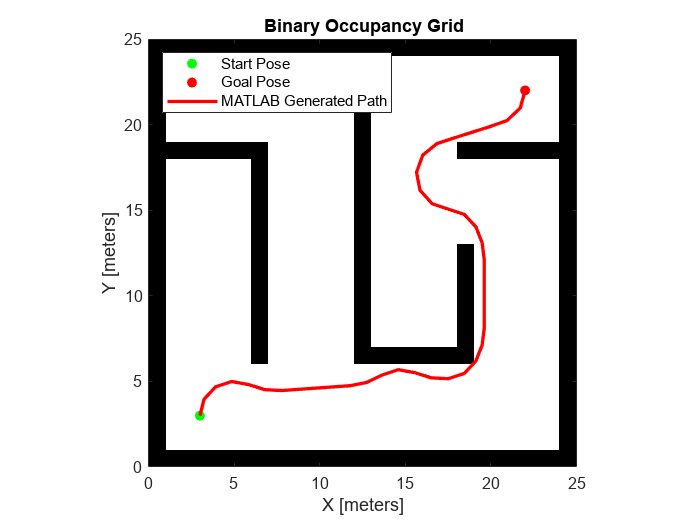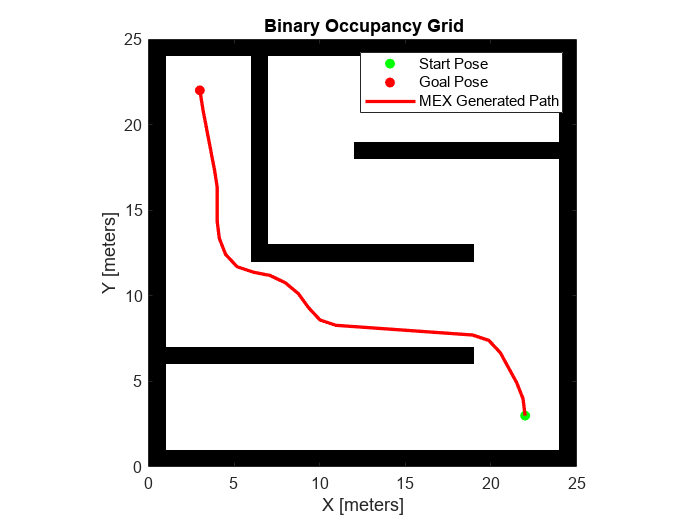# Generate Code for Path Planning Using Hybrid A Star

This example shows how to perform code generation to plan a collision-free path for a vehicle through a map using the Hybrid A* algorithm. After you verify the algorithm in MATLAB®, use the generated MEX file in the algorithm to visualize the planned path.

### Write Algorithm to Plan Path

Create a function, `codegenPathPlanner`, that uses a `plannerHybridAStar` object to plan a path from the start pose to the goal pose in the map.

```function path = codegenPathPlanner(mapData,startPose,goalPose) % Copyright 2021 The MathWorks, Inc. %#codegen % Create a state space object stateSpace = stateSpaceSE2; % Create a binary occupancy map binMap = binaryOccupancyMap(mapData); % Construct a state validator object using the statespace and map object validator = validatorOccupancyMap(stateSpace,'Map',binMap); % Set the validation distance for the validator validator.ValidationDistance = 0.01; % Assign the state validator object to the plannerHybridAStar object planner = plannerHybridAStar(validator); % Compute a path for the given start and goal poses pathObj = plan(planner,startPose,goalPose); % Extract the path poses from the path object path = pathObj.States; end ```

This function acts as a wrapper for a standard Hybrid A* path planning call. It accepts standard inputs and returns a collision-free path as an array. Because you cannot use a handle object as an input or output of a function that is supported for code generation, create the planner object inside the function. Save the `codegenPathPlanner` function in your current folder.

### Verify Path Planning Algorithm in MATLAB

Verify the path planning algorithm in MATLAB before generating code.

Load a map into the workspace.

`mapData = load("exampleMaps.mat").simpleMap;`

Create a state space object.

`stateSpace = stateSpaceSE2;`

Create a binary occupancy map.

`binMap = binaryOccupancyMap(mapData);`

Construct a state validator object using the statespace and map object.

`stateValidator = validatorOccupancyMap(stateSpace,'Map',binMap);`

Set the validation distance for the validator.

`stateValidator.ValidationDistance = 0.01;`

Initialize the `plannerHybridAStar` object with the state validator object.

`planner = plannerHybridAStar(stateValidator);`

Define start and goal poses as [x y theta] vectors. x and y specify the position in meters, and theta specifies the orientation angle in radians.

```startPose = [5 5 pi/2]; goalPose = [22 4 0];```

Plan a path from the start pose to the goal pose.

`plan(planner,startPose,goalPose);`

Visualize the path using the `show` function, and hide the expansion tree.

```show(planner,Tree="off") hold on```### Generate Code for Path Planning Algorithm

You can use either the `codegen` (MATLAB Coder) function or the MATLAB Coder (MATLAB Coder) app to generate code. For this example, generate a MEX file by calling `codegen` at the MATLAB command line. Specify sample input arguments for each input to the function using the `-args` option and `func_inputs` input argument.

Call the `codegen` function and specify the input arguments in a cell array. This function creates a separate `codegenPathPlanner_mex` function to use. You can also produce C code by using the `options` input argument. This step can take some time.

`codegen codegenPathPlanner -args {mapData,startPose,goalPose}`
```Code generation successful. ```

### Verify Results Using Generated MEX Function

Plan the path by calling the MEX version of the path planning algorithm for the specified start pose, and goal pose, and map.

`path = codegenPathPlanner_mex(mapData,startPose,goalPose);`

Visualize the path computed by the MEX version of the path planning algorithm.

```scatter(path(:,1),path(:,2),... Marker="o",... MarkerFaceColor="b",... MarkerEdgeColor="b") legend("MATLAB Generated Path","Start Pose","Goal Pose","MEX Generated Path") hold off```### Check Performance of Generated Code

Compare the execution time of the generated MEX function to the execution time of your original function by using `timeit`.

`time = timeit(@() codegenPathPlanner(mapData,startPose,goalPose))`
```time = 0.1287 ```
`mexTime = timeit(@() codegenPathPlanner_mex(mapData,startPose,goalPose))`
```mexTime = 0.0187 ```
`time/mexTime`
```ans = 6.8671 ```

In this example, the MEX function runs more than five times faster. Results may vary for your system.

### Plan Path in New Map Using Generated MEX Function

Plan a path for a new start and goal poses in a new map. The size of the new map must be the same as the map used to generate the MEX function.

```mapNew = load("exampleMaze.mat").simpleMaze; startPoseNew = [10 8 pi]; goalPoseNew = [5 22 0]; pathNew = codegenPathPlanner_mex(mapNew,startPoseNew,goalPoseNew);```

Visualize the new path computed by the MEX function.

```show(binaryOccupancyMap(mapNew)) hold on scatter(pathNew(:,1),pathNew(:,2),... Marker="o",... MarkerFaceColor="b",... MarkerEdgeColor="b")```